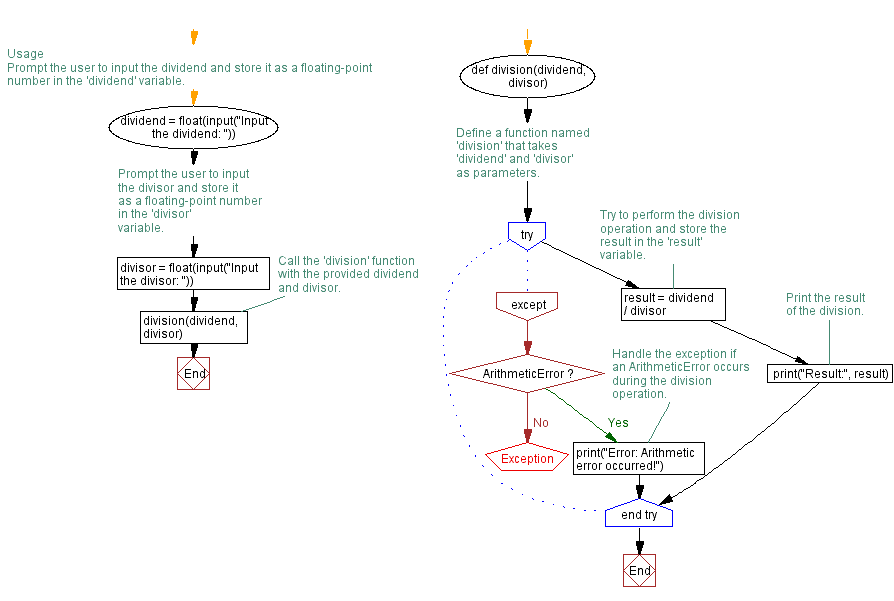﻿ Python program for Handling Arithmetic errors

# Handling ArithmeticError exception in Python division program

## Python Exception Handling: Exercise-8 with Solution

Write a Python program that executes division and handles an ArithmeticError exception if there is an arithmetic error.

exception ArithmeticError:

The base class for those built-in exceptions that are raised for various arithmetic errors: OverflowError, ZeroDivisionError, FloatingPointError.

Sample Solution:

Code:

``````# Define a function named 'division' that takes 'dividend' and 'divisor' as parameters.
def division(dividend, divisor):
try:
# Try to perform the division operation and store the result in the 'result' variable.
result = dividend / divisor
# Print the result of the division.
print("Result:", result)
except ArithmeticError:
# Handle the exception if an ArithmeticError occurs during the division operation.
print("Error: Arithmetic error occurred!")

# Usage

# Prompt the user to input the dividend and store it as a floating-point number in the 'dividend' variable.
dividend = float(input("Input the dividend: "))
# Prompt the user to input the divisor and store it as a floating-point number in the 'divisor' variable.
divisor = float(input("Input the divisor: "))
# Call the 'division' function with the provided dividend and divisor.
division(dividend, divisor)
``````

Explanation:

In the above exercise -

• The "perform_division()" function takes two parameters: dividend and divisor, representing the numbers to be divided.
• Inside the function, we use a try block to perform the division operation. If the division can be performed without arithmetic error, the result is assigned to the result variable.
• The result is then printed.
• If an arithmetic error occurs during division, such as dividing by zero, an ArithmeticError exception is raised. We catch the ArithmeticError exception in the except block and print an error message indicating that an arithmetic error occurred.

Output:

```Input the dividend: 12
Input the divisor: 4
Result: 3.0
```
```Input the dividend: 12
Input the divisor: 0
Error: Arithmetic error occurred!
```

Flowchart:What is the difficulty level of this exercise?

Test your Programming skills with w3resource's quiz.

﻿